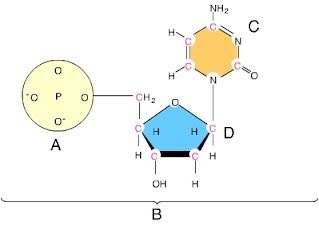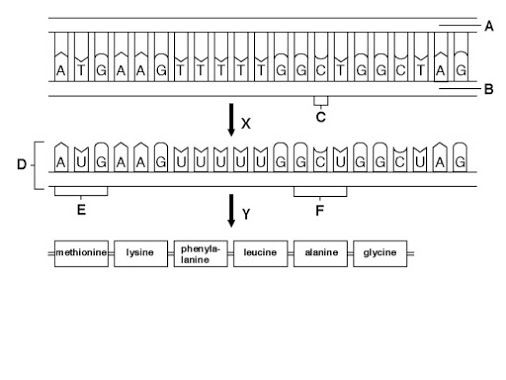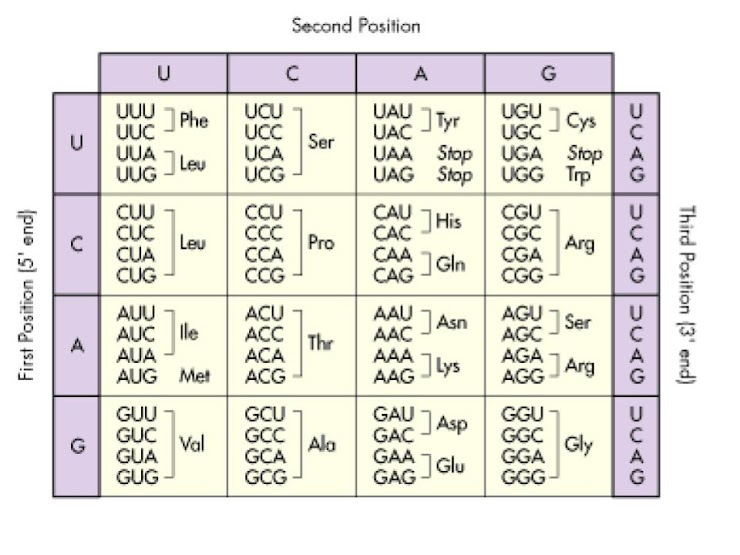DNA transcription/translation 2019 Agbio HONORS
Email *
Last Name *
First Name *
period *
1. An RNA nucleotide COULD be composed of following parts. check all that apply.
2 points
3. What are the two steps in reading the DNA code? check all that apply
2 points
4. If a DNA strand reading 3' TTT GAG CCC ATA 5' is transcribed, what mRNA would you make?
2 points
5. What are the 3 types of RNA. Check all that apply
2 points
Diagram 26. If diagram 2 above were a RNA nucleotide, letter C could NOT be
2 points
7 . In a sample of DNA, 25% of the nitrogenous bases are thymine. The complementary strand would consist of
2 points
8. The main enzyme (s) involved in TRANSCRIPTION is (are) CHECK ALL THAT APPLY
2 points
9. In diagram 3 below, X represents
2 points10. In diagram 3 below, Y represents
2 points11. In the diagram below, molecule A is
2 points12. In diagram below molecule D is
2 points13. According to Hank in the video HOT POCKETS that you were asked to watch, the molecule Titin is
2 points
14. What mRNA would we make if we transcribed the following piece of DNA: 3' AAA GCT GTA 5'
2 points
15. Before mRNA can leave the nucleus, it first must be
2 points
16. A messenger RNA molecule for making a protein is made in the nucleus and sent out to a ribosome. The ribosome reads the mRNA and makes a protein containing 120 amino acids. The mRNA consisted of at least how many codons?
2 points
17. The nucleotide sequence of DNA is GTA. A messenger RNA molecule with a complementary codon is transcribed from the DNA. In the process of translation (protein synthesis), a transfer RNA pairs with the mRNA codon. What is the base sequence of the tRNA anticodon?
2 points
18. Using the diagram below (diagram 3), what portion of the protein molecule is coded for the the following mRNA? mRNA = CUC AAG UGC UUC
2 points